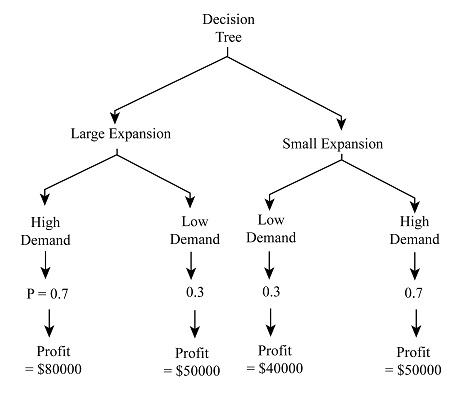# The owners of Sweet-tooth Bakery have determined that they need to expand their facility in order...

## Question:

The owners of Sweet-tooth Bakery have determined that they need to expand their facility in order to meet their increased demand for baked goods. The decision is whether to expand now with a large facility or expand small with the possibility of having to expand again in five years. The owners have estimated the following chances for demand:

The likelihood of demand being high is 0.70.

The likelihood of demand being low is 0.30.

Profits for each alternative have been estimated as follows: Large expansion has an estimated profitability of either $80,000 or$50,000, depending on whether demand turns out to be high or low.

Small expansion has a profitability of $40,000, assuming demand is low. Small expansion with an occurrence of high demand would require considering whether to expand further. If the bakery expands at this point, the profit-ability is to be$50,000.

(a) Draw a decision tree showing the decisions, chance events, and their probabilities, as well as the profitability of outcomes.

(b) Solve the decision tree and decide what the bakery should do.

## Decision Tree

Decision Tree is the representation of all the alternatives which are possible for the organisation to choose but all the alternatives have different expected payoff. The decision tree helps to determine which alternative can be chosen.

(a)

The decision tree given below showing all the alternatives:(b)

{eq}{\rm{Expected}}\,{\rm{Payoff}} = ({\rm{x}} \times {\rm{P}}\,{\rm{of}}\,{\rm{high}}\,{\rm{demand}}) - {\rm{y}} \times {\rm{P}}\,{\rm{of}}\,{\rm{low}}\,{\rm{demand}}) {/eq}

Large Expansion:

{eq}\begin{align*} {\rm{Expected}}\,{\rm{Payoff}} &= (\$80000 \times 0.7) - (\$ 50000 \times 0.3)\\ &= \$56000 - \$ 15000\\ &= \41000 \end{align*} {/eq} Small Expansion: {eq}\begin{align*} {\rm{Expected}}\,{\rm{Payoff}} &= (\ 50000 \times 0.7) - (\$40000 \times 0.3)\\ &= \$ 35000 - \$12000\\ &= \$ 23000 \end{align*} {/eq}

Conclusion: The expected payoff is high in large expansion. Therefore, it should be selected, that is, the company should largely expand the bakery at the present only.